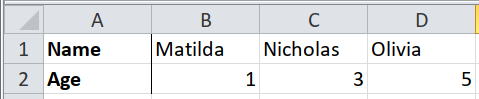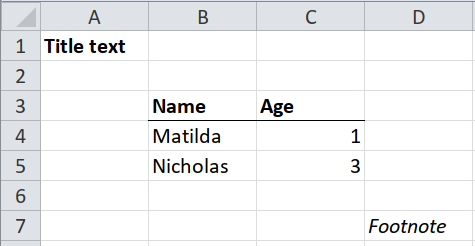## 2.2 Almost-tidy tables

For tables that are already ‘tidy’ (a single row of column headers), use packages like readxl that specialise in importing tidy data.

### 2.2.1 Transposed (headers in the first row, data extends to the right)Most packages for importing data assume that the headers are in the first row, and each row of data is an observation. They usually don’t support the alternative: headers in the first column, and each column of data is an observation.

You can hack a way around this by importing without recognising any headers, transposing with `t()` (which outputs a matrix), placing the headers as names, and converting back to a data frame, but this almost always results in all the data types being converted.

``````path <- system.file("extdata", "worked-examples.xlsx", package = "unpivotr")
read_excel(path, sheet = "transposed", col_names = FALSE) %>%
t() %>%
`colnames<-`(.[1, ]) %>%
.[-1, ] %>%
as_tibble()``````
``````## New names:
## * `` -> ...1
## * `` -> ...2
## * `` -> ...3
## * `` -> ...4``````
``````## # A tibble: 3 x 2
##   Name     Age
##   <chr>    <chr>
## 1 Matilda  1
## 2 Nicholas 3
## 3 Olivia   5``````

Tidyxl and unpivotr are agnostic to the layout of tables. Importing the transpose is the same is importing the usual layout, merely using the `"left"` direction instead of `"up"` when beheading the headers.

``````xlsx_cells(path, sheet = "transposed") %>%
select(col, data_type, header, character, numeric) %>%
select(Name, Age)``````
``````## # A tibble: 3 x 2
##   Name       Age
##   <chr>    <dbl>
## 1 Matilda      1
## 2 Nicholas     3
## 3 Olivia       5``````

### 2.2.2 Other stuff on the same sheetIt will be more complicated when the table doesn’t begin in cell A1, or if there are non-blank cells above, below or either side of the table.

If you know at coding time which rows and columns the table occupies, then you can do the following.

• Blank or non-blank cells above the table: use the `skip` argument of `readxl::read_excel()`.
• Blank or non-blank cells either side of the table: use the `col_types` argument of `readxl::read_excel()` to ignore those columns.
• Blank or non-blank cells below the table: use `n_max` argument of `readxl::read_excel()` to ignore those rows.
``````path <- system.file("extdata", "worked-examples.xlsx", package = "unpivotr")
sheet = "notes",
skip = 2,
n_max = 33,
col_types = c("guess", "guess", "skip")) %>%
drop_na()``````
``````## # A tibble: 2 x 2
##   Name       Age
##   <chr>    <dbl>
## 1 Matilda      1
## 2 Nicholas     3``````

If you don’t know at coding time which rows and columns the table occupies (e.g. when the latest version of the spreadsheet is published and the table has moved), then one strategy is to read the spreadsheet with `tidyxl::xlsx_cells()` first, and inspect the results to determine the boundaries of the table. Then use those boundaries as the `skip`, `n_max` and `col_types` arguments to `readxl::read_excel()`

1. Read the spreadsheet with `tidyxl::xlsx_cells()`. Filter the result for sentinel values, e.g. the cells containing the first and final column headers, and a cell in the final row of data.
2. Construct the arguments `skip`, `n_max` and `col_types` so that `readxl::read_excel()` gets the exact dimensions of the table.
``````# Step 1: read the spreadsheet and filter for sentinel values to detect the
# top-left and bottom-right cells
cells <- xlsx_cells(path, sheet = "notes")
rectify(cells)``````
``````## # A tibble: 7 x 5
##   `row/col` `1(A)`     `2(B)`   `3(C)` `4(D)`
##       <int> <chr>      <chr>    <chr>  <chr>
## 1         1 Title text <NA>     <NA>   <NA>
## 2         2 <NA>       <NA>     <NA>   <NA>
## 3         3 <NA>       Name     Age    <NA>
## 4         4 <NA>       Matilda  1      <NA>
## 5         5 <NA>       Nicholas 3      <NA>
## 6         6 <NA>       <NA>     <NA>   <NA>
## 7         7 <NA>       <NA>     <NA>   Footnote``````
``````top_left <-
dplyr::filter(cells, character == "Name") %>%
select(row, col)
top_left``````
``````## # A tibble: 1 x 2
##     row   col
##   <int> <int>
## 1     3     2``````
``````# It can be tricky to find the bottom-right cell because you have to make some
# assumptions.  Here we assume that only cells within the table are numeric.
bottom_right <-
dplyr::filter(cells, data_type == "numeric") %>%
summarise(row = max(row), col = max(col))
bottom_right``````
``````## # A tibble: 1 x 2
##     row   col
##   <int> <int>
## 1     5     3``````
``````# Step 2: construct the arguments `skip` and `n_max` for read_excel()
skip <- top_left\$row - 1L
n_rows <- bottom_right\$row - skip

read_excel(path, sheet = "notes", skip = skip, n_max = n_rows)``````
``````## # A tibble: 2 x 2
##   Name       Age
##   <chr>    <dbl>
## 1 Matilda      1
## 2 Nicholas     3``````

Here’s another way using only tidyxl and unpivotr.

``````# Step 2: filter for cells between the top-left and bottom-right, and spatter
# into a table
cells %>%
dplyr::filter(between(row, top_left\$row, bottom_right\$row),
between(col, top_left\$col, bottom_right\$col)) %>%
select(row, col, data_type, character, numeric) %>%
``````## # A tibble: 2 x 2